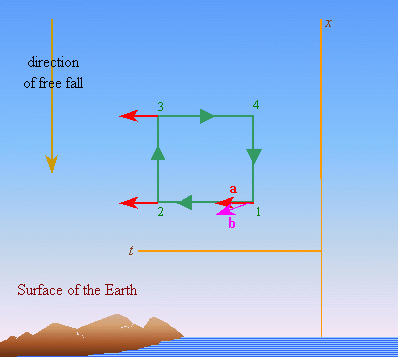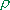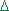V.6 No 1 63 The problem of physical time in today physicsViolating this principle, relativists have to seek the curvature of space with the clearly non-obvious methods. As we saw in the above citation from Schwarzschild, he wrote a trivial expression of the curved 4-D surface (5.3). At the same time, as we saw in case of material body on the curved surface, we plotted this surface in the larger coordinate system. This is trivial for the Riemann geometry: “in the general Riemann geometry accepted in our (relativistic – Authors) theory as the basis, gany ten functions of the four coordinates xcan exist. The 4-D continuum that obeys the Riemann geometry can be ‘graphically’ shown as the surface of four dimensions drawn in the Euclidean hyperspace of quite large number of dimensions. Factually, it needs 10 dimensions – according to the quantity g” [7, p. 280]. Thus, it appears that leaving the Euclidean space, relativists never left it, as the very curvature of Riemann geometry has to reveal itself in some space of higher dimension, and the metric of this covering space has, nonetheless, to be Euclidean, not Riemann. If the dimension of Riemann space is 4, the very fact of curvature has to reveal in relation to the space of more dimensions. Just so Eddington, when having determined the nesting of the Riemann geometry into the Euclidean space of higher dimension, immediately reserved: “When we use the expression ‘curvature’ for the space-time, we always think this last located just so in the Euclidean space of higher dimension. We do not want to say so that such space of higher dimension exists; the aim of such visualisation is only to more clearly imagine the metric properties of the world” [7, p. 281]. In this way he factually admitted that the problem with the space curvature on the account of material entities exists. But one thing is – to reduce the non-physical representation of the space-time curvature to the reservations expecting that “the 4-D surface able to freely curve in six additional dimensions has incredible scope of abilities” [7, p. 281], and basically other thing is to determine experimentally this curvature in supposition that the (3+1)-dimensional physical space which relativists curve has the same dimensionality as the space into which it is nested. This is what Bergmann tried to do in his book “The riddle of gravitation”: “Apart from dividing the curvature into components, it is important to know the value characterising the curvature as the whole and determining the specific physical situation. Instead the turn angle related to the unit of area – normal measure of curvature (as it requires to increase the dimensionality of space – Authors), we can select some other measure, closer to our intuition, for example, sphere whose surface has a given curvature. The less is sphere the more its curvature. The unit of curvature is a surface on which the vector of unit length turns by one radian, when it is transferred along the boundary of quadrate with the unit side… Now let us pass to the gravity field on the Earth surface… Choose now a rectangular as a closed-loop path for the parallel transfer in a freely falling frame; one pair of its reciprocal sides is spatially-like and vertical, the length of each side is 1 m and is in parallel to the axis x; the second pair of sides is time-like, horizontal, their ‘length’ is 1 s. If one of two time-like sides we put by its one end into the origin of freely falling frame, another time-like side will be at a distance of 1 m from the origin, where the imaginary gravity acceleration will be equal to 1,610-6 m/s2” [48, p. 86–87]. Let us stop here to think about Bergmann’s statement of the difference in the acceleration at the time-like sides. First of all, introducing such difference to the acceleration of free fall, Bergmann ‘forgot’ his earlier statement that “in the free falling frame we cannot see inertial accelerations, nor accelerations of gravitation (gravity accelerations)” [48, p. 78]. Proceeding from this, Bergmann had no right to say of change of things that cannot be detected in the frame of his choice, although he said: “vice versa, the presence of gravity field produces such phenomena in the inertial reference frame that locally cannot distinct from the inertial acceleration which we could see in a non-inertial reference frame” [48, p. 79]. This dual interpretation of possibility to measure the acceleration of free fall brings Bergmann to a conclusion that “The gravity acceleration is roughly 10 m/s2, and this is just the acceleration of freely falling frame relative to Earth (! – Authors) … As the Earth radius is about 6000 km, the acceleration of relatively freely falling reference frame (! – Authors) varies with the ‘speed’ of 10 m/s2 per 6000 km of distance from the start place, i.e. with the ‘speed’ of 1,610-6 s –2 ” [48, p. 87]. True, if “the acceleration of relatively freely falling reference frame varies with the ‘speed’ of 10 m/s2 per 6000 km”, there has to be a body in relation to which this value would vary at least kinematically. And this body has to rest in relation to the gravitating body, the measurements of non-kinematic nature to be accurate without application of Newton laws. As the gravitating body (in this case the Earth) is not under acceleration by premise, the body by whose motion Bergmann is about to measure the free fall acceleration is also inertial. But when relativists speak of curving, they necessarily pass to the freely falling frame, as according to the relativistic understanding, “The procedures targeted to reveal the inertial frame in an unlimited area of space and for an unlimited interval of time are far from being unambiguous, and we see no ways to make them unambiguous” [48, p. 79]. So “Einstein expressed pro to reject any attempts restoring the previous part of inertial reference frames. Locally their part has to be passed to the freely falling frames” [48, p. 79]. And he said so when, after just a relativistic statement, “Among the frames that allow to be continued, no hierarchy is possible; no criterion to select a class of special or privileged frames. All reference frames have to be considered as equivalent” [48, p. 79]. We will also note for further consideration that on one hand Bergmann, following Einstein, introduces a frame freely falling in the gravity field as some universal frame in which the equivalence of laws of nature is true, and on the other hand he states the gravity field inhomogeneous in it, in order to reveal the curvature in this frame. This nuance will be crucially important for us in the analysis of equivalence of laws of nature in inertial and non-inertial frames which, as we already said, we will do in the next section. Here we will confine ourselves to the statement of fact that without change of strength of the gravity field, which means – without the value of free-fall acceleration at the time-like sides of Bergmann’s loop, he is unable to reveal the curvature of space-time, and we will see it from his following consideration. Continuing the analysis, how Bergmann states the problem, we can see that, if Bergmann decided to determine the curvature in this way, he has to be interested in the value of acceleration, not only in the affection of difference of acceleration at the second time-like side of the loop that he took. Actually, according to the technique that Bergmann describes, “Let now the vector a be transferred in parallel along this rectangle (see Fig. 5.4 – Authors): we will choose the vector a parallel to the time axis t – such will be the vector of speed of the resting trial body. A parallel transfer along the time axis does not change a least this vector (in relation to the freely falling frame), as the trial body that initially rested will remain at rest. The transfer along the space-like side also will bring no changes, but the transfer along the third time-like side will cause the change of speed by the value 1,610-6 m/s2, therefore we yield the vector b. The transfer along the fourth space-like side will change nothing, again” [48, p. 87–88].Fig. 5.4. The curvature of the gravity field of Earth [48, p. 88, Fig. 40]
 As we can see from this citation, irrespectively of, whether Bergmann measures the Gaussian curvature of 4-D multiplicity either the radius of equivalent sphere, he has to form the closed loop and to determine the variation of vector in tracing this loop; with it, in absence of difference of accelerations at the time-like sides of loop, i.e. in the homogeneous gravity field, the curvature of space-time after Bergmann has to vanish. In abstract geometry, where the axes of multi-dimensional multiplicity are not identified with the physical parameters and, consequently, are abstracted from their specific properties, such operation of loop tracing does not raise problems. But geometric constructions in physics are sensible only in case when they account the physical properties; violation of this rule causes absurds and paradoxes. We see it in Bergmann’s construction, too. Noting the physical features of space and time, in this problem Bergmann may not transfer an abstract vector along the loop. He has to transfer a trial body to which he can attribute some vector of speed, or this ‘vector’ that does not relate to any material substance will not fall synchronously with the frame. But what it means – to transfer along the spatial part of loop the time-like vector together with the material body? As Bergmann says, at the section 1–2 of the loop the body rests in its frame. With it, the vector of speed is directed with the time axis. At the section 3–4 the body rests again and the vector again (at least at the point 3) is directed along the time axis. Between the points 2 and 3 the body passes along the spatial axis, but with it, after Bergmann, the vector of speed remains time-like. This is basically impossible, irrespectively of, with which speed this time-like vector will be transferred. To transfer a body from one spatial point to another, we necessarily have to add to the body some speed, which would change the operation of transfer, as well as the result of this transfer will be other. This discrepancy follows from the above described relativistic tearing geometry away from the experience and as a consequence – from use of geometric transforms out of limits in which they are correct. Geometry considers only spatial objects and, we can repeat, “In the strongly logical reasoning in proving theorems we have to deal only with these properties of objects” [31, p. 37]. So when in differential geometry someone says of n-D dynamic multiplicity, the time determines exceptionally the shift of curvilinear surfaces. In particular, “Absolutely same as for orthogonal systems on the surface, we can take the parameter (! – Authors) t as the time and to understand the equalities … as the equations of motion of a particle of liquid with the initial curvilinear coordinates,1 and2 . In such case the stationary flow of liquid transferring the surfaces of three-orthogonal system S serves as the mechanical treatment of the group of transforms” [51, p. 211]. Thus, the time in this case is accounted namely so as this is done in classical physics when we find the shift of three-orthogonal system by way of linear either nonlinear transforms. But if, in accordance with relativistic understanding, the time multiplied by the speed of light takes an equivalent place in the Riemann multiplicity: “coordinates are identifying numbers attributed to the points of space-time. Between the quantitative measure and identifying numbers there is no distinction, so we can think the change of coordinates as a particular case of general change applied to all quantitative measures. The coordinate variation already will not take such selected part…; now it already is equivalent to other changes of measures” [7, p. 86], – as we said above, this violates the main property of time – its irreversibility that makes the basic distinction of time from the spatial coordinates. We immediately see it in Bergmann’s diagram. Let at some moment  t1  the trial body is at the point 1 of the loop. It will arrive to the point 2 at the moment t2 = t1 + 1   (in seconds); to the point 3 it will arrive in some time which is necessary, the material body to pass along the given path; in connection with the above said, we disregard that Bergmann’s side 2–3 is perpendicular to the time axis – we base on the statement of relativists that it is impossible to transfer the material body faster than the speed of light: “From the law of speeds addition there follows also another interesting corollary: there cannot be an interaction which we could use to transmit signals and which propagates faster than light in the void” [52, p. 76]. Let it be the moment t3 . Now, noting that we go the loop round sequentially, let us think: at which moment of time the body will arrive to the point 4? At the moment t4 = t3 + 1 ? No, we go round the path 3–4 in the negative direction of the time axis. This means, according to the construction t4 = t3 - 1  and noting sequential times, we will yield t1 < t4 < t2 . But during this interval of time between  t1  and t2  the body was at the segment 1–2! Thus, according to Bergmann, going sequentially round his loop, the material body has to be located simultaneously at two spatial points of freely falling coordinate system. And not only in Bergmann’s problem. In all cases, be it a specific model after Bergmann or abstract formalism of Einstein or his followers, any attempt to form a closed loop involving the time parameter will bifurcate the material substance in space. This evidences that the very identification of time with the spatial coordinates is incorrect, and we may not substitute it by the numerical measures. The time has specific physical properties, it disallows such identification. This makes Einstein’s 4-D representation false, because it is based on the incorrect, from the view of physical nature, interpretation of geometric abstraction. Relativists used this practice from the very beginning, when they joined the time and spatial metrics into one mutually dependent continuum. Basically, they did so not on the grounds that joined so different parameters describing the physical processes but on the illegal generalisation of the particular case of light beam propagation that has only indirect relation to the concept of time. And “The statement that the light passes distances AM and BM at one and same time factually is not the premise either hypothesis of the physical nature of light, it is the statement which we can do on the grounds of free choice, to come to the definition of simultaneity” [50, p. 542]. So factually relativists did not join the concepts of space and time, as they state it – they voluntarily and without grounds ‘agreed’ with themselves the constant speed of light in all reference frames, irrespectively of the properties of real space and time, and on the basis of this ‘agreement’ together with the ‘agreement’ of the full identity of all inertial (and non-inertial) frames, they founded their conception, forcing the experimenters to seek just this foreshortening in which their compromising constructions would seem to be trustworthy. On this way, as we showed it in , they corrupted the initial concepts being the basis of Maxwellian theory, and, as we said above, changed the meaning of equivalence of the reference frames, squeezing all these concepts under corrupted mathematical nucleus of transforms of differential geometry. So nothing of surprise that relativists yielded paradoxical results. One of such results was the inclined plane of simultaneous events in the Lorentz transforms. Judging by the above citation, the introduced equality of times when the beam passes ahead and back in the inertial frame served to introduce the simultaneity. As the result of these efforts, “the differencet'  of times of two events relative to K' , generally speaking, does not vanish even when the difference of timest   of these events relative to K disappears. To the merely spatial distance of two events relative to the frame K, there relates the distance in time of the same events relative to K' ” [50, p. 558–559]. Thus, introducing their conditions of simultaneity, relativists violated the principle of simultaneity. Moreover, as we showed in , the Lorentz transforms not only incline the plane of events but the degree of time transformation depends on the direction and speed of the object relative to this frame, which makes the transforms generally unreal, as well as unreal is the 4-D multiplicity on which relativists based their general theory of relativity.

Contents: / 53 / 54 / 55 / 56 / 57 / 58 / 59 / 60 / 61 / 62 / 63 / 64 / 65 / 66 / 67 / 68 / 69 / 70 / 71 / 72 / 73 / 74 / 75 / 76 / 77 / 78 / 79 / 80 / 81 / 82 / 83 /